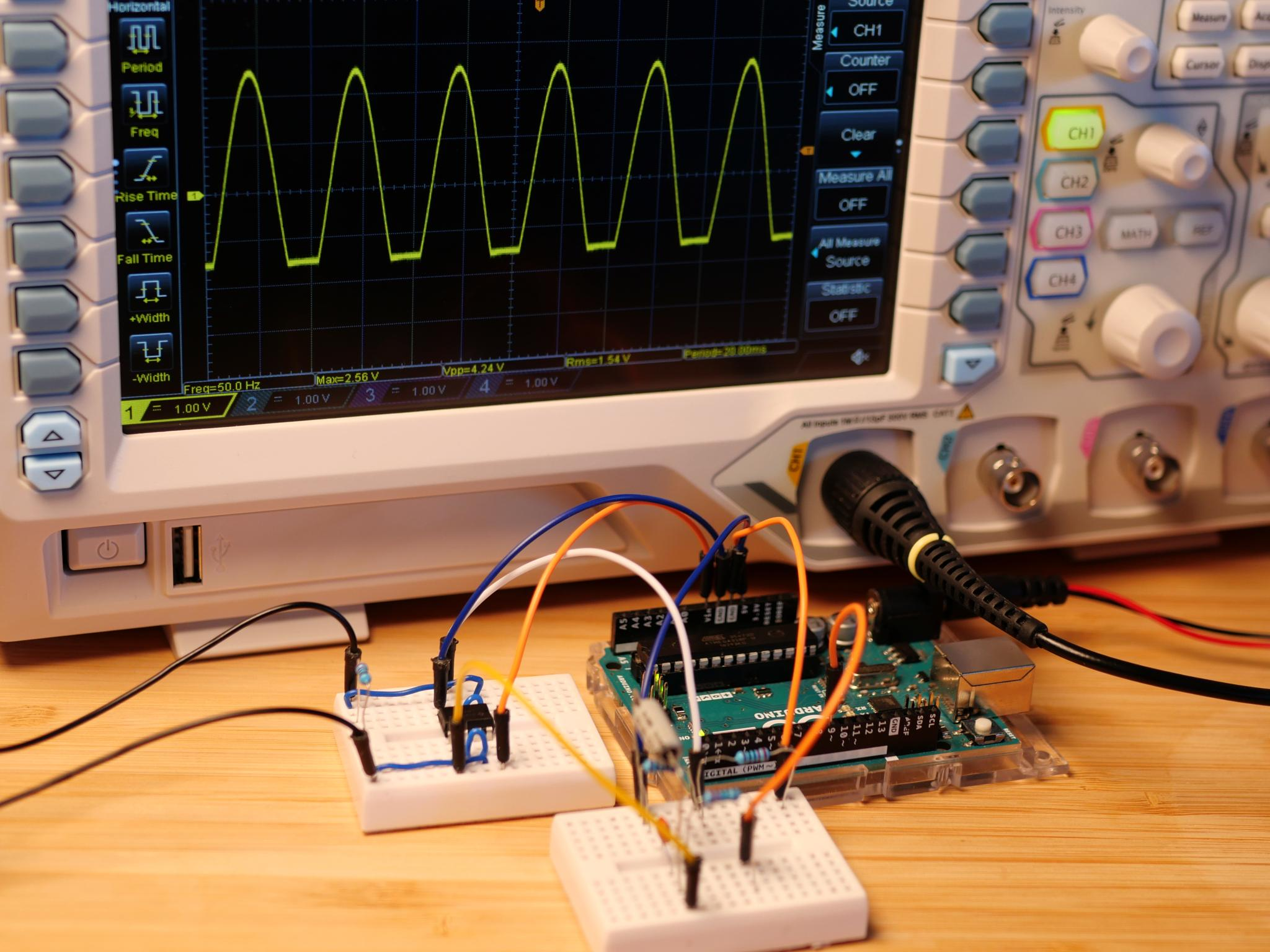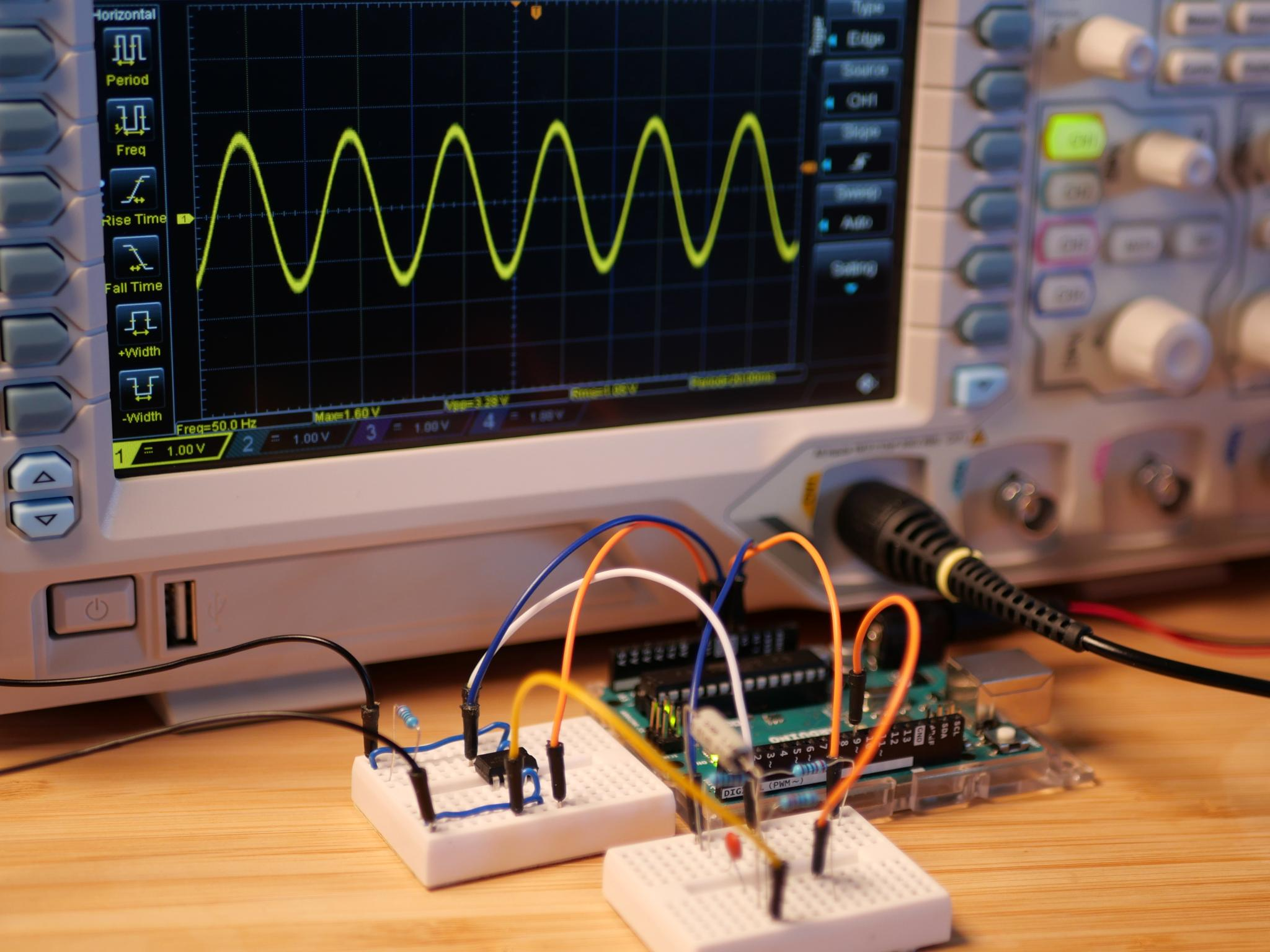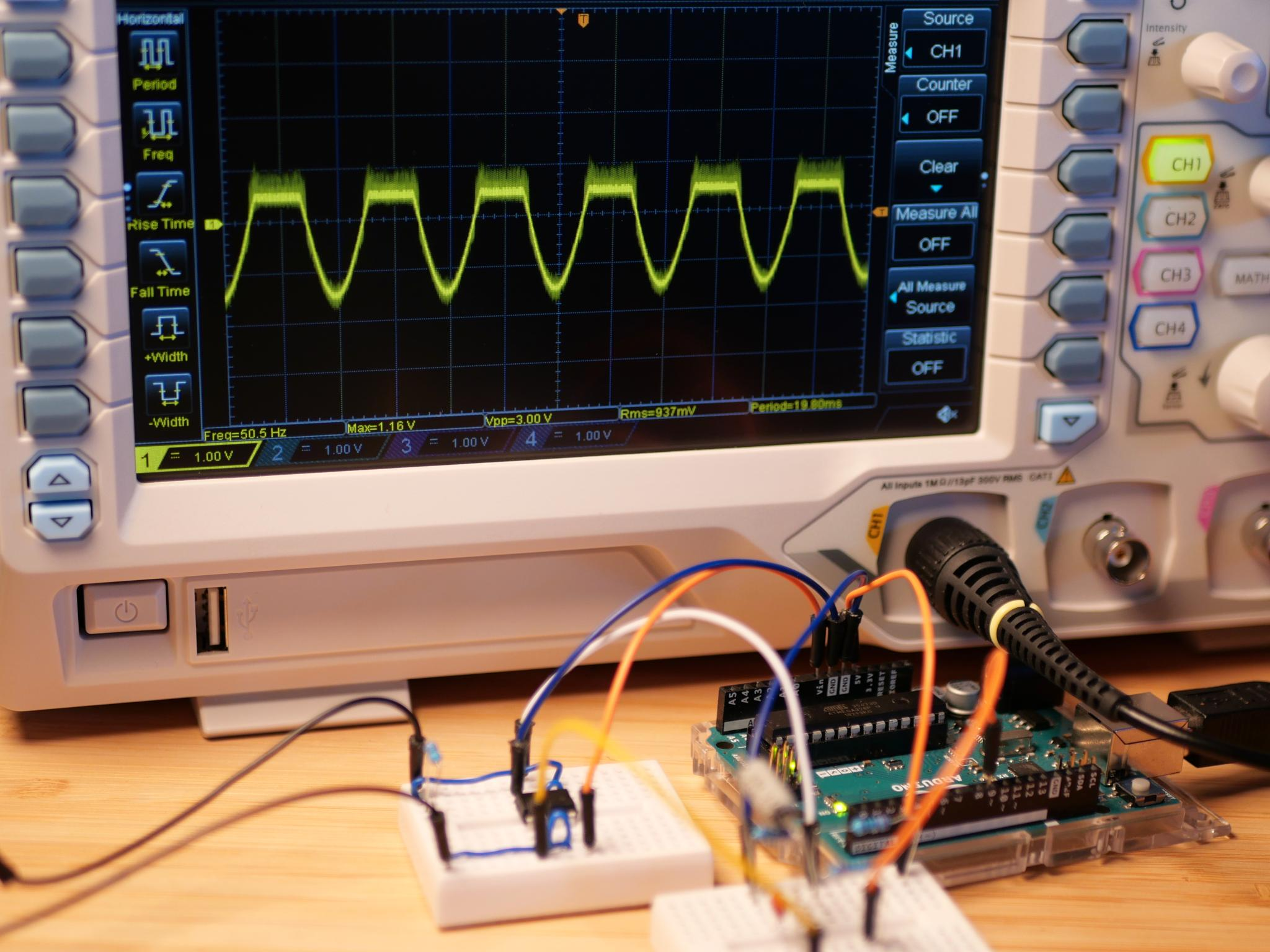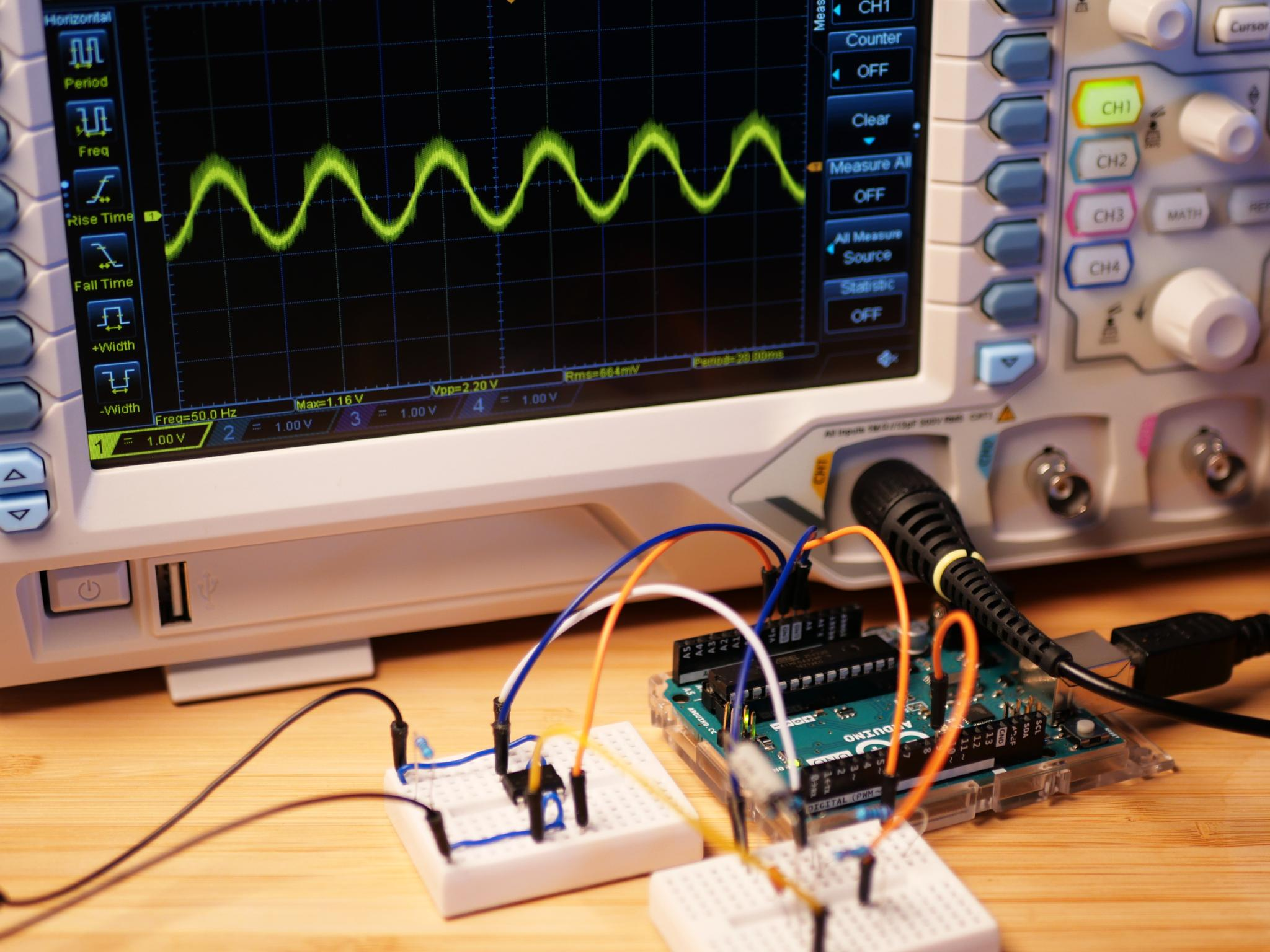# Low Voltage AC Source (Part 5)

After we learned about different ways to generate a sine wave, it is now time to speak about amplifying the output signal.

## Amplification

In the last parts of this project we looked at different ways to generate a sine wave. All these solutions shared a common issue: the generated signal becomes distorted or unstable under load. For this reason, we always used a high resistance load of 10 kΩ. Now, it is time to change that and speak about amplification.

There are different ways to amplify a signal. In case of analog signals, however, using an operational amplifier (op amp) is probably the most common method. In our case, we are going to use an LM358 as amplifier. Before we start right way, we should however define what exactly we are going to amplify. There are three different possibilities: we can increase the power, the maximum output current or the voltage. In this part of our project, the focus is on stabilizing the output signal and ensuring, that the sine wave signal is outputted properly even in case of a low resistance load. In other words, we aim for an increased output current.

## Operational Amplifiers

Before we look at the actual amplification circuit, let's talk about operational amplifiers (op amps) first. Operational amplifiers are differential amplifiers. They amplify the voltage difference between the two input pins IN+ and IN-. Op amps typically have a really high gain. In case of the LM358 the voltage difference is amplified by a factor of roughly 100 000. The exact factor is voltage, frequency and temperature dependent. Of course, the output voltage of an operational amplifier is never bigger than it's supply voltage. In fact, the maximum output voltage is often around 1.5V below the supply voltage. The reason for this is that op amps typically use transistors for amplification. Silicon transistors need a base-emitter voltage of around 0.7 V before they start conducting. Rail-to-rail op amps are specifically designed to be able to output a voltage up to the supply voltage. They are, however, more complex and thus of course more expensive. This is, however, just a side note, as the LM358, that we are using here is not a rail-to-rail op amp anyway.

What's the point in using an operational amplifier, if the high voltage gain causes the output signal to be either the maximum or the minimum possible voltage? Well, one possible application is to produce a digital signal that signals whether the voltage at IN+ is higher than the one at IN-. However, for this application there exist specialized op amps, which are called comparators. In most cases, op amps are not used in the so-called open-loop configuration where the full amplification factor applies. In the image below you can see another op-amp circuit: a so-called voltage buffer.In a voltage buffer circuit the op amps output is connected to the IN- input of the amplifier. Using an output signal as an input signal at the same time is called a feedback loop. The amplifiers output directly affects the amplifiers behavior as it is used as input too. Let's see how this affects the behavior of the op amp.

As already said, the op amp amplifies the voltage difference between the inverting input IN- and the non-inverting input IN+. If the output is connected to IN-, this means that voltage at the output is identical to the one at the non-inverting input. In case the output voltage is lower than the input voltage at IN+, there is a positive voltage difference, which is amplified and causes the output voltage to rise. In case the voltage at IN-is higher, we have a negative voltage difference and the output voltage decreases. The stable state that is reached after a short period of time is the one, in which the voltage at both inputs is equal. In this state the output voltage is exactly the same as the input voltage at IN+. A voltage buffer circuit has a voltage gain of 1, which is also called unity gain. The voltage is not amplified, however, the maximum output current is now defined by the limits of the op amp instead of the ones of our signal generating circuit. We thus achieve a current gain as desired.

## Amplifying the Output Current

Let's move on and talk about how we are going to use the op amp in our circuit. The LM358 contains two op amps. We are going to use both of them in voltage buffer configuration. One of them is going to be used to amplify the sine wave signal and the other one to amplify the 2.5 V, we use as ground reference. We can use this basic amplification circuit in conjunction with all the previous signal generation circuits. For this we simply connect the output of the signal generations circuit to the input of the amplifier circuit.### Building the Amplifier Circuit

Let's look at how we can apply this idea to the two solutions that worked best: using a PWM output or the external MCP4725 module as DAC. The image below shows, how to build up the amplifier circuit on a breadboard and combine it with the signal generation circuit.Be aware that I used a 9 V battery as power supply. These 9 V are used to power the amplifier which then can achieve a theoretic maximum output voltage of up to 7.5 V. If we used the 5V of the Arduino, the maximum output voltage would be 3.5 V, which would be bad since, we are trying to amplify a signal between 0 V and 5 V. If you still want to build the circuit without the external 9 V battery, have a look at the circuit proposed in the next section.

Does this circuit work out of the box without any code modification? Well, in theory it should and indeed it does, but only as long as no load is connected. As soon as the 220 Ω load resistor is connected, the output signal looks like this:Why does this happen? Wasn't the point of amplifying the signal, that the output signal remains stable under load? Well, this is only true for a certain voltage range. For the negative half-wave the current flows from the 2.5 V ground reference into the op amp that amplifies the sine wave signal. The op amp operates as current sink. However, even if the minimum output voltage for the LM358 is 0 V, it cannot sink a notable amount current at this voltage, just like it can't source current close to the supply voltage. Once again, the reason for this is, that the op amp is using a transistor to connect the output to ground. This doesn't work as soon as the output voltage drops below a certain value and this is why our signal is cut-off at the bottom.

There a two ways to solve this. Similar to connecting the op amps VIN pin to 9 V we could connect the op amps ground pin to a voltage below 0 V. This is not a working option for us, as we don't have a voltage below the Arduino's 0 V. The alternative is to adjust the amplitude of the generated sine wave, so that the output voltage never drops below the critical value. For this we are going to reduce the peak-to-peak voltage to 3 V which means that the lowest voltage is 1 V above the Arduino's ground. This corresponds to -1.5 V in reference to our artificial 2.5 V ground reference. At 1V the op amp should be capable of sinking a current of roughly 10 mA. This is not much, but far better than the non-amplified version, where we started to run into problem at a few milliamperes. The required code adjustment is shown below:

// Precalculate Sine Values between 1 V - 4 V (51 - 204)
for(int i = 0; i < steps; i++) {
voltages[i] = sin(i*3.14*2/steps) * 76.5 + 127.5;
}

After this adjustment the output signal is a perfect sine wave, even if the 220 Ω load is connected:### An Alternative Circuit for 5 V

Is there a way to make this circuit work without the 9V battery? Well, if we just keep the circuit and code used before, the output signal looks like this:Without the 9 V battery, the output voltage range is limited even further. The new usable voltage range is from 1 V to roughly 2.7 V. Above this voltage the current that can be supplied during the positive half-wave is not high enough for the 220 Ω load. With this new voltage range, it doesn't make sense to use 2.5 V as midpoint anymore. The real mid between 1 V and 2.7 V would be 1.85 V. By replacing the 220 Ω resistor to the Arduino's ground with a 120 Ω resistor, we can create a voltage of roughly 1.75 V, which is close enough to 1.85 V and doesn't require a fancy resistor value. We can then generate a sine wave with an amplitude of 0.75 V in the voltage range between 1 V and 2.5 V.

Below you can see the adjusted circuit and code:// Precalculate Sine Values between 1 V - 2.5 V (51 - 127)
for(int i = 0; i < steps; i++) {
voltages[i] = sin(i*3.14*2/steps) * 38.25 + 89.25;
}

With these changes, we once again get a complete sine wave signal, although with a much lower amplitude:## The Result

As we could see, we were able to stabilize the generated sine wave signal by amplifying the current. This way we can now use a 220 Ω load instead of 10 kΩ. The output signal remains stable until a current of roughly 10 mA. At higher currents the sine wave gets clipped at the top and the bottom, as the op amp is not capable to source and especially sink more current. To sum up, it's only a small improvement and our result still not great.

A possible solution to further improve the circuit is using an additional push-pull stage with more powerful transistors. However, this would mean further voltage losses, which is a real no-go regarding our already very limited output voltage range. So before we look into this, we should speak about amplifying the voltage. This is going to be the topic for the next part of this project.